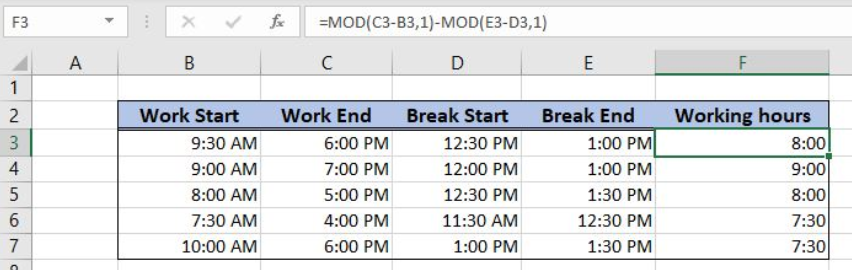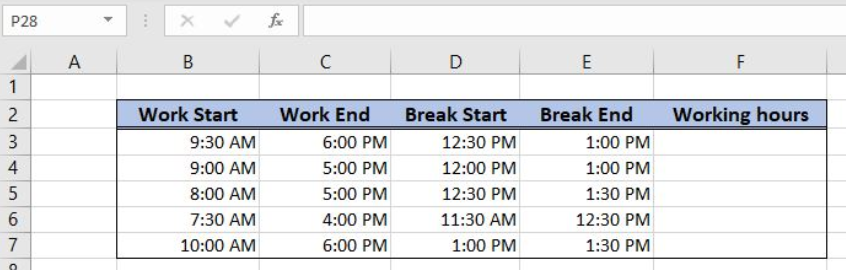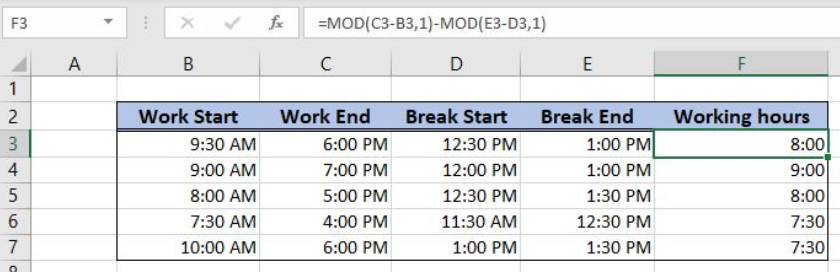Get instant live expert help with Excel or Google Sheets“My Excelchat expert helped me in less than 20 minutes, saving me what would have been 5 hours of work!”

#### Post your problem and you’ll get Expert help in seconds.

Your message must be at least 40 characters
Our professional Expert are available now. Your privacy is guaranteed.

# Basic timesheet formula with breaks

Excel allows us to get create a basic timesheet formula with breaks using the MOD function. This step by step tutorial will assist all levels of Excel users in creating a basic timesheet formula with breaks.Figure 1. The result of the MOD function

## Syntax of the MOD Formula

`=MOD(number, divisor)`

The parameters of the MOD function are:

• number – a number which we want to divide
• divisor – a divisor, in our example 1.

## Setting up Our Data for the MOD Formula

Let’s look at the structure of the data. Our table consists of 5 columns. The first two are “Work Start” (column B) and “Work End” (column C). The next two columns are “Break Start” (column D) and “Break End” (column E). In the column F (“Working hours”) we want to get working hours, excluding breaks.Figure 2. The data structure for the MOD example

## Calculating the Basic Timesheet Formula with Breaks Using the MOD Function

In the cell F3, we want to get working hours as a difference between work start and work and (B3 and C3) minus break length (a difference between break start and break end – D3 and E3).

The formula looks like:

`=MOD(C3-B3, 1) - MOD(E3-D3, 1)`

The first MOD function has number C3-B3 and divisor 1, while the second has number E3-D3 and divisor 1. When these two numbers are subtracted, we get the working hours.

To apply the MOD function we need to follow these steps:

• Select cell F3 and click on it
• Insert the formula: `=MOD(C3-B3, 1) - MOD(E3-D3, 1)`
• Press Enter
• Drag the formula down to the other cells in the column by clicking and dragging the little “+” icon at the bottom-right of the cell.Figure 3. Create the basic timesheet formula with breaks using the MOD function

The first MOD function returns 0.3541666 and the second one 0.0208333. When these two values are subtracted the result is 0.333333, which is equal to 8 hours in the cell F3.

Most of the time, the problem you will need to solve will be more complex than a simple application of a formula or function. If you want to save hours of research and frustration, try our live Excelchat service! Our Excel Experts are available 24/7 to answer any Excel question you may have. We guarantee a connection within 30 seconds and a customized solution within 20 minutes.

### Did this post not answer your question? Get a solution from connecting with the expert.Another blog reader asked this question today on Excelchat:
Solution examplesI need help with this formula. I need this to include months =INT((M4)/7)&" weeks"&IF(MOD(M4,7)=0,"",", "&INT(MOD(M4,7))&" days") I have the number of Days to Start with
Solved by F. A. in 29 minsneed help with the different sheets and formula IF(A4=B4,0,NETWORKDAYS(A4+1,B4,\$E\$9:\$E\$42) +0.5*(INT((+B4-A4+1)/7)+IF(WEEKDAY(A4+1)+MOD (B4-A4-1,7)>=7,1,0)))
Solved by O. D. in 11 minsi have this formula currently which works but not if I want it for every day - any ideas ?? (NETWORKDAYS.INTL(L1362,M1362,11,BH!A:A)-1)*("17:30"-"8:30")+IF(NETWORKDAYS.INTL(M1362,M1362,11,BH!A:A),MEDIAN(MOD(M1362,1),"8:30","17:30"),"17:30")-MEDIAN(NETWORKDAYS.INTL(L1362,L1362,11,BH!A:A)*MOD(L1362,1),"8:30","17:30")
Solved by A. D. in 28 mins## Subscribe to Excelchat.coAnother blog reader asked this question today on Excelchat: